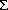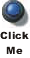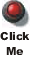# PHY138Y - Mechanics - Class 8 - Wed. Oct. 6, 2004

## Introduction

Newton was very bothered by the action-at-a-distance which is part of his law of universal gravitation. He justified it by saying that it worked, and that he would rely on future physicists to deal with this. In fact, he almost dealt with it himself. The following, from the Principia shows that he was getting pretty close to the concept of a gravitational field:

The gravitational forces are "an endeavor of the whole directed toward a center, ... a certain efficacy diffused from the center through each of the surrounding spaces."

## Announcements

Pre-Class Quiz #4 (WebCT) released.

• Due Wed. Oct. 13 by 10 AM.
• Sections 7.4 - 7.7 and Chapter 8

MP Problem Set #4 released.

• Due Fri. Oct 15 by 5 PM
• Chapter 7

## Work-Kinetic Energy Theorem

The text derives the work-kinetic energy theorem using some integral calculus which, as we discussed in the last class, we don't expect you to know about yet. In class we derived the theorem for the case of a constant force. Since this is not in the text, I repeat that derivation here:

First, we write the work for a constant force F acting on an object of mass m over a distance x.

W = F · x

If F is in the direction of x, then:

W = F x

From Newton's Second Law this is:

W = ma x

But, as we learned in Chapter 2, average acceleration is defined as:

a = ( vf - vi ) /t

We also learned in Chapter 2 that for constant acceleration the distance is the average speed times the time:

x = ( vf + vi ) /2 * t

So the work is:

W = m ( vf - vi ) /t ( vf + vi ) /2 * t = 1/2 m vf 2 - 1/2 m vi 2

So, if we define the kinetic energy K as:

K = 1/2 m v2

Then we have proved that for a constant force:

W =K

The text proves that the above relation is true for any force.

## Vis Viva

Contemporaneously with Newton, not only Robert Hooke but Gottfried Leibniz was wrestling with the problem of forces, accelerations, and gravity. He realised that in many collisions the quantity:mv2

was conserved. He named this quantity vis viva, or "the living force."

Now we use a variation of this idea. We say that the quantity:1/2 mv2

is conserved, and we call it the kinetic energy. This is, of course, just one-half of the vis viva.

## In-Class Question

 We asked a question about Racing Balls, and did a demonstration. Initially about 1/4 of the class chose 1) Ball A and 3/4 chose 3) They reach the end of the track at the same time. Only a few chose 2) Ball B.After discussing it amongst yourselves for a couple of minutes the class was nearly unanimous that the answer is 3) They reach the end of the track at the same time. We did the demonstration, which showed that the correct answer is 2) Ball B.

 The button to the right accesses a Flash animation that duplicates the demonstration we used in class.If you are a skier, you might find the Flash animation accessed with the button to the right helps you think about this problem.## A Bit of History of the Concept of Energy

Prior to the early 1800's most people believed that heat was some sort of fluid that flowed from one body to the other; the fluid was called caloric. People attempted to measure its mass by measuring the increase in mass of a body when it was heated. It was only later that it was realised the heat was just another form of energy. In those days the unit of heat was the calorie.

In the document accessed below in the Basal Metabolism section we will discuss this history a bit more.

The laboratory has an experiment that is very similar to a classic experiment done by Joule in 1847 to measure how much energy is contained in a calorie of heat. The experiment will be available to you in the 2nd term, and is called The Mechanical Equivalent of Heat. The guide sheet for the experiment is available at:

Here is a perhaps apocryphal story about Joule. He married and went to a resort for his honeymoon. The resort had a waterfall. He was so fascinated by the identification of heat as just another form of energy that he spent a large amount of time measuring the difference in temperature of the water and top and the bottom of the waterfall. This caused his wife to leave him. So the "honeymoon" was over before the honeymoon was over.

## Textbook Mistake

In §6.8 the textbook has a mistake. It states that the British unit of power is the horsepower: it is actually ft-lb/sec. The horsepower is a derived unit, due to James Watt in 1775: he noticed that "a strong horse could lift 150 pounds a height of 220 feet in 1 minute." The defines one horsepower. It is equal to:

150 lb x 220 feet / 1 minute x 1 minute/60 seconds = 550 ft-lb/sec.

## Basal Metabolism

 We discussed an application of conservation of energy, and introduced an extension of the dimensional analysis of Chapter 1. Notes on this have been prepared.